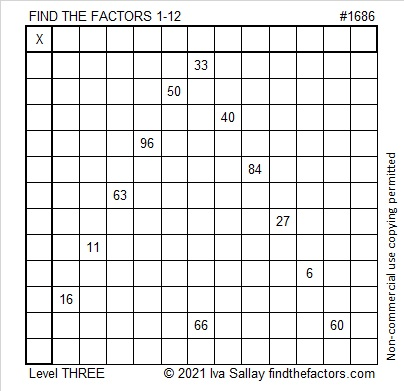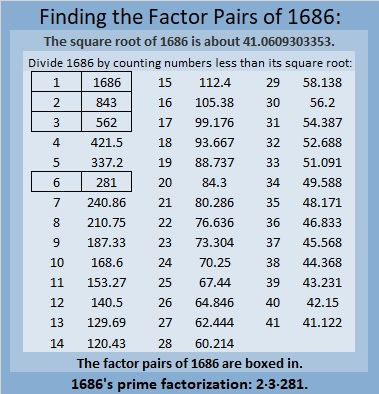# 1686 Some Candy Corn for You to Chew on

### Today’s Puzzle:

Candy corn probably isn’t your favorite Halloween treat, but this candy corn puzzle could give you something satisfying to chew on. Give it a try!

Find the common factor of 33 and 66, write the factors in the appropriate cells. Since this is a level 3 puzzle, you can then work from the top of the puzzle row by row until you have found all the factors. The numbers from 1 to 12 must appear once in both the first column and the top row.Here’s the same puzzle without any color if you prefer:### Factors of 1686:

• 1686 is a composite number.
• Prime factorization: 1686 = 2 × 3 × 281.
• 1686 has no exponents greater than 1 in its prime factorization, so √1686 cannot be simplified.
• The exponents in the prime factorization are 1, 1, and 1. Adding one to each exponent and multiplying we get (1 + 1)(1 + 1)(1 + 1) = 2 × 2 × 2 = 8. Therefore 1686 has exactly 8 factors.
• The factors of 1686 are outlined with their factor pair partners in the graphic below.### More about the number 1686:

1686 is the hypotenuse of a Pythagorean triple:
960-1386-1686, which is 6 times (160-231-281).

1686 is also a leg in these two Pythagorean triples:
1686-710648-710650, calculated from 2(843)(1), 843² – 1², 843² + 1² and
1686-78952-78970, calculated from 2(281)(3), 281² – 3², 281² – 3².